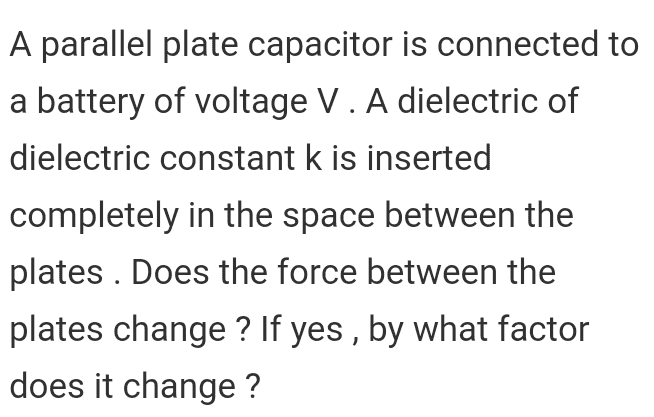# Dielectric inserted in parallel plate capacitor

Jahnavi

## Homework Statement## The Attempt at a Solution

The force between the plates is given by (1/2)QE ,where Q is the charge on the plates and E is the electric field between the plates .

Now Q = CV and E = V/d , where C is the capacitance , V is the potential difference and d is the plate separation . So , force can be written as F = (1/2)(CV)(V/d) .When dielectric is inserted C changes to "kC" .V doesn't change as the plates are connected to battery .

So , force F increases by a factor "k'" to become kF on insertion of the dielectric while plates are connected to battery .

Is my work correct ?

#### Attachments

Homework Helper
Gold Member
The force between the plates is given by (1/2)QE ,where Q is the charge on the plates and E is the electric field between the plates .
I think you need to be careful with calling E the electric field between the plates when there is a dielectric between the plates. The force on Q of one of the plates depends on the value of the field at the surface of the plate where Q is located. Is this value of E the same as the value of the field inside the dielectric? For instance, consider the case where there is a thin layer of vacuum between each plate and the dielectric material. In this case, the field at Q would be the field in the vacuum layer between the plate and the dielectric. This would be true no matter how thin you make the vacuum layer.

•Jahnavi
Jahnavi
So , if E is the electric field at the surface of plates and E' is electric field between the plates , then E' = E/k and V = E'd .This gives E = Vk/d .

Force F = (1/2)(CV)(E) .After inserting the dielectric , C changes to kC and E could be written as Vk/d . So , basically force increases by a factor k2 .

Is that correct ?

Homework Helper
Gold Member
So , if E is the electric field at the surface of plates and E' is electric field between the plates , then E' = E/k and V = E'd .This gives E = Vk/d .

Force F = (1/2)(CV)(E) .After inserting the dielectric , C changes to kC and E could be written as Vk/d . So , basically force increases by a factor k2 .

Is that correct ?
I think that's correct.

•Jahnavi
Jahnavi
I think it is not wise to use the expression F = (1/2)QE .Instead F = Q2/2Aε0 looks easier to analyse . Using Q= CV , when battery is connected and dielectric is inserted , C increases by a factor k and so does the charge Q . Clearly F increases by a factor k2 .

In case when battery is not connected Q remains same and so force remains unchanged .

Easier to analyse. Isn't it ?

Homework Helper
Gold Member
I think it is not wise to use the expression F = (1/2)QE .Instead F = Q2/2Aε0 looks easier to analyse . Using Q= CV , when battery is connected and dielectric is inserted , C increases by a factor k and so does the charge Q . Clearly F increases by a factor k2 .

In case when battery is not connected Q remains same and so force remains unchanged .

Easier to analyse. Isn't it ?
Yes. That's a nicer way to look at it.

•Jahnavi
Jahnavi
Thanks !